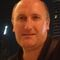Study Notes

# The Multiplier - Short Question Answers

Level:
AS, A-Level, IB
Board:
AQA, Edexcel, OCR, IB, Eduqas, WJEC

Last updated 9 Jan 2021

Here are some explanations for a small selection of questions on the multiplier.

### Question 1

In a closed economy with no government, the equilibrium level of income is £22 billion, the full employment level of income is £25 billion. To reach full employment would require an injection of £1 billion.What can be deduced from this information?

A closed economy means that we ignore the effects of exports and imports in the circular flow model.

No government means that we ignore government spending and taxation

Therefore the only injection is investment (I) and the only leakage is saving (S)

The economy is currently £3 billion below full-employment level of national income.

We are told that an injection of £1 billion is needed to close this gap.

Multiplier = final change in national income / initial injection of aggregate demand

Therefore the size of the national income multiplier must be 3

The formula for the simple multiplier is 1/MPS or 1/(1-MPC)

MPC + MPS = 1

If the multiplier is 3 then the marginal propensity to save must be 1/3 and the marginal propensity to consume must be 2/3

### Question 2

In a closed economy, the marginal propensity to save increases and tax rates remain unchanged. What effect will this have on the marginal propensity to consume and on the multiplier?

In a closed economy we ignore exports and imports. The only two leakages are saving and taxation and the two injections are investment and government spending.

The formula for the multiplier will be 1/marginal rate of withdrawal

I.e. 1/MPS + MRT

Assume the marginal propensity to save (MPS) = 0.3 and the marginal rate of tax (MRT) = 0.2

Therefore the multiplier = 1 / (0.3 + 0.2) = 2

If the marginal propensity of save to increases to 0.4 and the marginal rate of tax remains the same at 0.2

Therefore the multiplier = 1/ (0.4+0.2) = 1.67

I.e. the multiplier falls when the marginal rate of leakage increases.

### Question 3

In a closed economy with no government, a £1 billion increase in investment leads to a £5 billion increase in consumption. What is the value of the marginal propensity to consume?

The formula for the multiplier in a closed economy with no government is 1/marginal propensity to save or 1/(1-marginal propensity to consume)

We can infer from the information that the value of the multiplier = 5

Therefore the marginal propensity to save must be 0.2

Because MPS + MPC always equals 1

Then the MPC = 1-0.2 = 0.8

• 7

### Revision Flashcards for A Level Economics Students

Resource Collection

• 4

### Synoptic Revision Mats

Resource Collection

### Question 4

The average propensity to consume in a country is 0.75 and the marginal propensity to consumeis 0.8. What is the value of the multiplier?

Assume here that we are dealing with a simple multiplier where M = 1/MPS

If the marginal propensity to consume is 0.8 then the marginal propensity to save = 0.2, therefore the multiplier = 1/0.2 = 5

We can ignore the information on the average propensity to consume as this is irrelevant to the multiplier calculation.

### You might also like### Energy Economics - Hornsea 2 becomes fully operational

31st August 2022### Government Funding and the Multiplier Effect - British Volt and the Gigafactory

23rd January 2022

### Explaining the Multiplier Effect

Study Notes15th July 2021

Topic Videos

### UK regions compete to host 'gigafactory' for electric car batteries

16th November 20207th July 2020

### The Fiscal Multiplier

Topic Videos

© 2002-2022 Tutor2u Limited. Company Reg no: 04489574. VAT reg no 816865400.